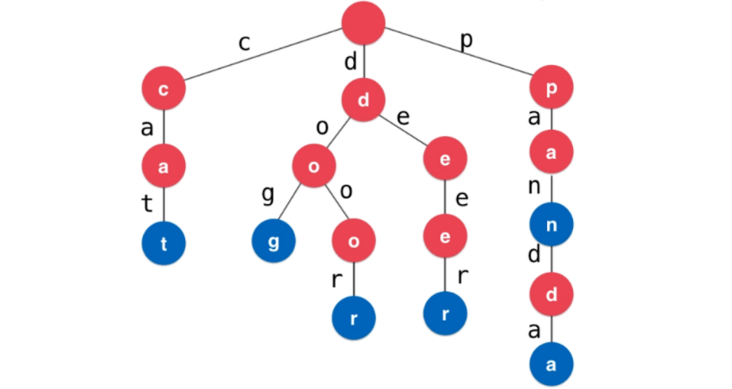## trie图 java_【数据结构】之字典树（前缀树、Trie）_宁输输的博客-程序员宅基地

1、什么是字典树import java.util.TreeMap;

/**

* 字典树、前缀树实现

*/

public class Trie {

/**

* 节点

*/

private class Node {

/**

* 是否是单词表示

*/

public boolean isWord;

/**

* 节点的子节点映射

*/

public TreeMap next;

public Node(boolean isWord) {

this.isWord = isWord;

next = new TreeMap<>();

}

public Node() {

this(false);

}

}

/**

* 根节点

*/

private Node root;

/**

* Trie树中存储的单词数量

*/

private int size;

/**

* 获取Trie中存储的单词数量

* @return

*/

public int getSize() {

return size;

}

/**

* 向Trie中添加一个新的单词Word

* @param word 单词

*/

Node cur = root;

for (int i = 0; i < word.length(); i++) {

char c = word.charAt(i);

if (cur.next.get(c) == null) {

cur.next.put(c, new Node());

}

cur = cur.next.get(c);

}

if (!cur.isWord) {

cur.isWord = true;

size++;

}

}

/**

* 判断Trie中是否包含单词Word

* @param word 待查询单词

* @return

*/

public boolean contain(String word) {

Node cur = root;

for (int i = 0; i < word.length(); i++) {

char c = word.charAt(i);

if (cur.next.get(c) == null) {

return false;

}

cur = cur.next.get(c);

}

return cur.isWord;

}

/**

* 判断Trie中是否包含前缀prefix

* @param prefix

* @return

*/

public boolean isPrefix(String prefix) {

Node cur = root;

for (int i = 0; i < prefix.length(); i++) {

char c = prefix.charAt(i);

if (cur.next.get(c) == null) {

return false;

}

cur = cur.next.get(c);

}

return true;

}

}

1、力扣677号问题(键值映射)

import java.util.TreeMap;

/**

* Your MapSum object will be instantiated and called as such:

* MapSum obj = new MapSum();

* obj.insert(key,val);

* int param_2 = obj.sum(prefix);

*/

class MapSum {

/**

* 节点

*/

private class Node {

/**

* 单词结束节点保存的值

*/

public int value;

/**

* 节点的子节点映射

*/

public TreeMap next;

public Node(int value) {

this.value = value;

next = new TreeMap<>();

}

public Node() {

this(0);

}

}

/**

* 根节点

*/

private Node root;

/** Initialize your data structure here. */

public MapSum() {

root = new Node();

}

/**

* 向Trie树中插入单词及其值

* @param word 单词

* @param val 值

*/

public void insert(String word, int val) {

Node cur = root;

for (int i = 0; i < word.length(); i++) {

char c = word.charAt(i);

if (cur.next.get(c) == null) {

cur.next.put(c, new Node());

}

cur = cur.next.get(c);

}

cur.value = val;

}

/**

* 前缀求和

* @param prefix

* @return

*/

public int sum(String prefix) {

Node cur = root;

for (int i = 0; i < prefix.length(); i++) {

char c = prefix.charAt(i);

if (cur.next.get(c) == null) {

return 0;

}

cur = cur.next.get(c);

}

return sum(cur);

}

private int sum(Node node) {

int res = node.value;

for (Character c : node.next.keySet()) {

res += sum(node.next.get(c));

}

return res;

}

}

1、基于TreeMap实现的字典树实现

### ffmpeg 录屏命令_ffmpeg录屏命令_越努力越幸运~的博客-程序员宅基地

window （安装dshow）ffmpeg -rtbufsize 100M -f dshow -i video="screen-capture-recorder":audio="virtual-audio-capturer" -vcodec libx264 -preset veryfast -crf 22 -tune:v zerolatency -pix_fmt yuv420p ..._ffmpeg录屏命令

### opensuse kde桌面配置 ibus输入法_opensuse切换ibus_迈向虚无的博客-程序员宅基地

opensuse kde桌面配置 ibus输入法ibus 输入法介绍ibus-anthy: 一个日文输入法引擎，基于anthy。ibus-pinyin: 该软件暂时没有维护，而且最新的 ibus 引擎上部分功能不能使用。作为替代，请使用ibus-libpinyin。ibus-rime:一个强大的智能中文输入法,支持拼音、注音或者没有音调的拼音、双拼、粤拼、中州韵、仓颉和五笔86。_opensuse切换ibus